# 2022 A-level H2 Mathematics (9758) Paper 2 Suggested SolutionsAll solutions here are suggested. Mr. Teng will hold no liability for any errors. Comments are entirely personal opinions

Question 1:Question 2: 12 seconds;m
Question 3: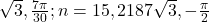Question 4:Question 5: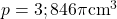Question 6:Question 7: Sample;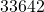Question 8:Question 9: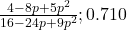Question 10:Question 11:; do not reject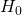•chun sheng

thanks for the solution, do you know if failing to remove anomalous pt in Q10 will cause all subsequent parts to be 0? since the r value, equation, calculated values would be different

•KS Teng

Hello, hope it doesn’t affect you. But you probably will lose the 2 marks in (f) and 1 mark in (g). The other 1 mark in (g) should be ok if you did explain that it is out of data range, etc.

•chun sheng

Thanks for the reply, just curious wouldn’t that be a triple penalty for the same mistake as I would have been already penalised in (d). Also, for (g) I explain that it is within data range as I did take into the anomalous pt. Would I be penalised again?

•KS Teng

But it is all answer mark and no method mark.

•tatiana

Hi! Thanks for ur solution 🙂 Could I check the mark allocation for Q 2,5,11 in terms of method marks?

•KS Teng

sorry, i do not recall the specific mark distribution. so it is difficult to comment.

•Rishabh

Hi, could I know how many marks is 7(a), 10(a) and 11(c)? Thanks!

•Rishabh

sorry 7(b) not 7(a)**

•KS Teng

sorry, i do not recall the actual marks distribution of them specifically. 11(c) should be 5.

•Hello, for the hypothesis testing question I got the same answer here but a lot of my classmates said that it is a new distribution, so the unbiased estimate and CLT should be used. Is this true?

Also for the last question last part, can I say an assumption is that the sample was chosen randomly?

•KS Teng

Generally we do know the population variance and question did specify it’s over a long time. Unbiased estimates can always be found and I guess they are also trying to test if students do read and consider the information given to them in exams. Being able to find the unbiased estimates does not imply population variance changes, the sample could be not representative.

•hello

So why did they specifically give 30 samples, which is the minium accepted value for a ‘large sample’? If you say they test on the student’s ability to read the question, then shouldn’t this detail be read too?
And, if you assume the distribution does not change, why the last part of the question ask you about 6 samples and assumptions? If ‘from reading the question’, we can already infer that the distribution after the change in training remains normal, then there is no reason to write that as an assumption, since it is ‘given’ already. However, if you are assuming it, means it is not given. Then your point on concluding that Zhou’s new distribution being ‘given’ as normal is wrong.

•KS Teng

If you refer to school notes, then its stated then given population variance, use it. else find the unbiased estimate of population variance. So if we know population variance, then there should not be a need to use the unbiased estimate.
In statistics, when we have a sample, we can definitely find the sample parameters and the unbiased estimates. But we are supposed to discern which parameters are meant to be used.

•Edward

But that part is about the person having a new training regime so shouldn’t variance change

•KS Teng

yes he is using a new regime, but he is testing if the population mean changed. the null hypothesis is testing if the population mean, 80 changed. we did not change this population mean to 79 either, due to finding an unbiased estimate of population mean. so we have to be clear about what are the population parameters, sample parameters and unbiased estimate of population parameters.

•john

8a shouldnt variance be +

•KS Teng

Yes my bad. Typo. Sorry bout it

•Johnson

For q11 isn’t it a different training regime. Then shouldn’t the variance be the unbiased estimate

•KS Teng

If we follow the hypothesis structure, then even school notes made it quite clear that if population variance is given for the normal distribution, then we should use population variance.

•Kim

I was wondering if the hypothesis test result should be “do not reject H0” since his timing did not go below 80. Thank you

•KS Teng

You should be rejecting since p value is less than level of significance

•Matthew

For q11 the hypo testing question, shouldn’t the new variance be used as its a new training regime

•KS Teng

If we follow the hypothesis structure, then even school notes made it quite clear that if population variance is given for the normal distribution, then we should use population variance.

•ya nao

hi, thanks for the solution! Do you know how many marks we would lose if we used gc and p-value for question 11?

•KS Teng

I dont think it is a problem so long as you did state the test statistic out. since with the test statistic, one can easily find the p value.

•H2 Math Genius

For question 8 (a) shouldnt you always add variance?

•KS Teng

•James

this was the worse paper i’ve ever done i’ll say my total % for math will be around 55% 😭😭 is that enough to get a B?

•landon

Hi man what do you think the % required for B is this year?? I messed up my papers and got roughly 55% is that enough 😭😭😭

Not readable? Change text.